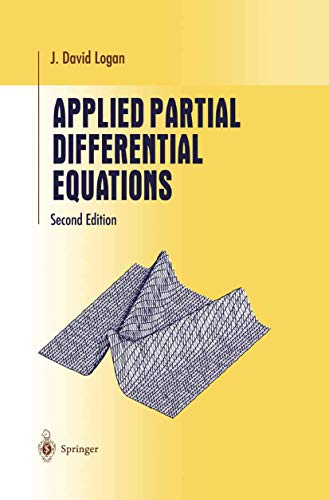# Applied Partial Differential Equations

## Logan, J. David

Valutazione media 3,62
( su 8 valutazioni fornite da Goodreads )Vedi tutte le copie di questo ISBN:

This text is written for the standard, one-semester, undergraduate course in elementary partial differential equations. The topics include derivations of some of the standard equations of mathematical physics (including the heat equation, the wave equation, and Laplace's equation) and methods for solving those equations on bounded and unbounded domains. Methods include eigenfunction expansions, or separation of variables, and methods based on Fourier and Laplace transforms.

Le informazioni nella sezione "Riassunto" possono far riferimento a edizioni diverse di questo titolo.

Recensione:

From the reviews of the second edition:

"This second edition of the short undergraduate text provides a fist course in PDE aimed at students in mathematics, engineering and the sciences. The material is standard ... . Strong emphasis is put on modeling and applications throughout; the main text is supplied with many examples and exercises." (R. Steinbauer, Monatshefte für Mathematik, Vol. 150 (4), 2007)

"This book contains an elementary introduction of partial differential equations to undergraduate students in mathematics, engineering, and physical sciences. ... This is a unique book in the sense that it provides a coverage of the main topics of the subject in a concise style which is accessible to science and engineering students. ... Reading this book and solving the problems, the students will have a solid base for a course in partial differential equations ... ." (Tibor Krisztin, Acta Scientiarum Mathematicarum, Vol. 74, 2008)

Dalla quarta di copertina:

This primer on elementary partial differential equations presents the standard material usually covered in a one-semester, undergraduate course on boundary value problems and PDEs. What makes this book unique is that it is a brief treatment, yet it covers all the major ideas: the wave equation, the diffusion equation, the Laplace equation, and the advection equation on bounded and unbounded domains. Methods include eigenfunction expansions, integral transforms, and characteristics. Mathematical ideas are motivated from physical problems, and the exposition is presented in a concise style accessible to science and engineering students; emphasis is on motivation, concepts, methods, and interpretation, rather than formal theory.

This second edition contains new and additional exercises, and it includes a new chapter on the applications of PDEs to biology: age structured models, pattern formation; epidemic wave fronts, and advection-diffusion processes. The student who reads through this book and solves many of the exercises will have a sound knowledge base for upper division mathematics, science, and engineering courses where detailed models and applications are introduced.

J. David Logan is Professor of Mathematics at University of Nebraska, Lincoln. He is also the author of numerous books, including Transport Modeling in Hydrogeochemical Systems (Springer 2001).

Le informazioni nella sezione "Su questo libro" possono far riferimento a edizioni diverse di questo titolo.

### Altre edizioni note dello stesso titolo

#### Edizione in evidenza

ISBN 10:  0387984399 ISBN 13:  9780387984391
Casa editrice: Springer-Verlag, 1998
BrossuraSpring..., 1998
RilegatoSpring..., 2004
Rilegato## 1.Applied Partial Differential Equations (Undergraduate Texts in Mathematics)

Editore: Springer
ISBN 10: 0387209530 ISBN 13: 9780387209531
Nuovo PAPERBACK Quantit�: 1
Da
Cloud 9 Books
(Wellington, FL, U.S.A.)

Descrizione libro Springer. PAPERBACK. Condizione: New. 0387209530 New Condition. Codice articolo NEW33.1809859

Compra nuovo
EUR 46,32
Convertire valuta
Spese di spedizione: EUR 4,49
In U.S.A.
Destinazione, tempi e costi

## 2.APPLIED PARTIAL DIFFERENTIAL EQU

ISBN 10: 0387209530 ISBN 13: 9780387209531
Nuovo Quantit�: 1
Da
BennettBooksLtd
(San Diego, CA, U.S.A.)

Descrizione libro Condizione: New. New. Codice articolo M-0387209530

Compra nuovo
EUR 85,17
Convertire valuta
Spese di spedizione: EUR 4,45
In U.S.A.
Destinazione, tempi e costi

## 3.Applied Partial Differential Equations (Undergraduate Texts in Mathematics)

Editore: Springer (2004)
ISBN 10: 0387209530 ISBN 13: 9780387209531
Nuovo Paperback Quantit�: 1
Da
Book Deals
(Lewiston, NY, U.S.A.)

Descrizione libro Springer, 2004. Paperback. Condizione: BRAND NEW. Codice articolo 0387209530_abe_bn

Compra nuovo
EUR 107,08
Convertire valuta
Spese di spedizione: GRATIS
In U.S.A.
Destinazione, tempi e costi